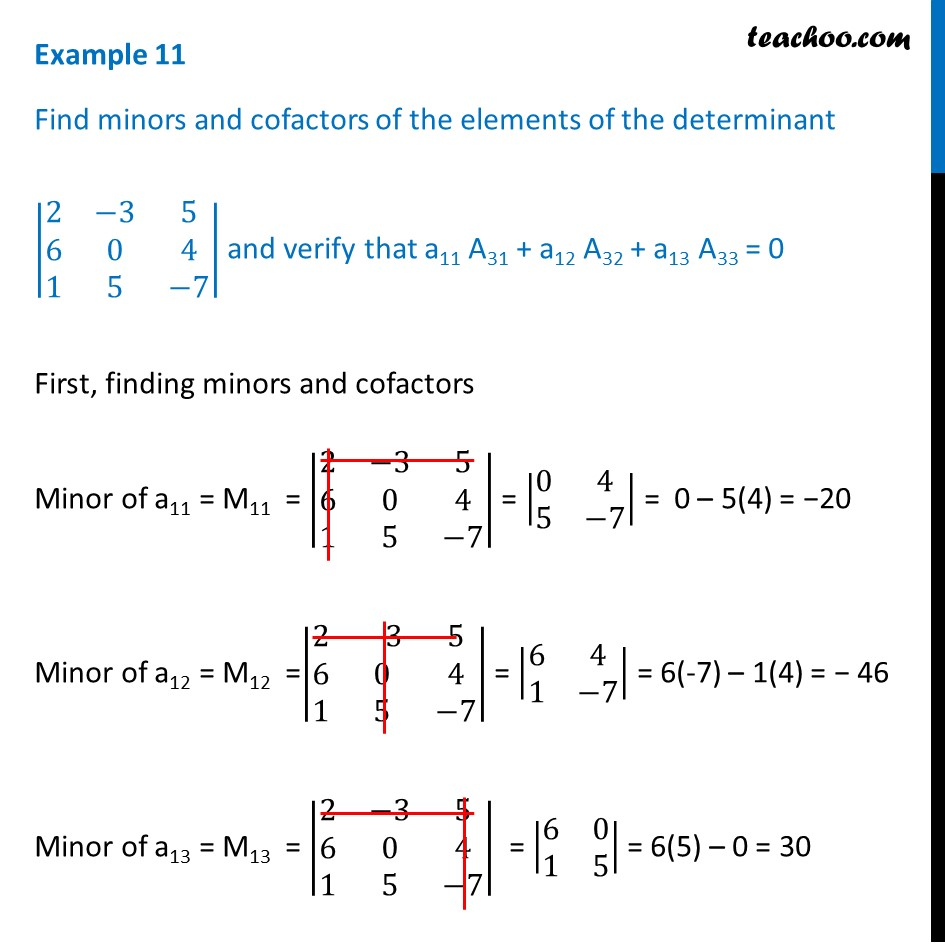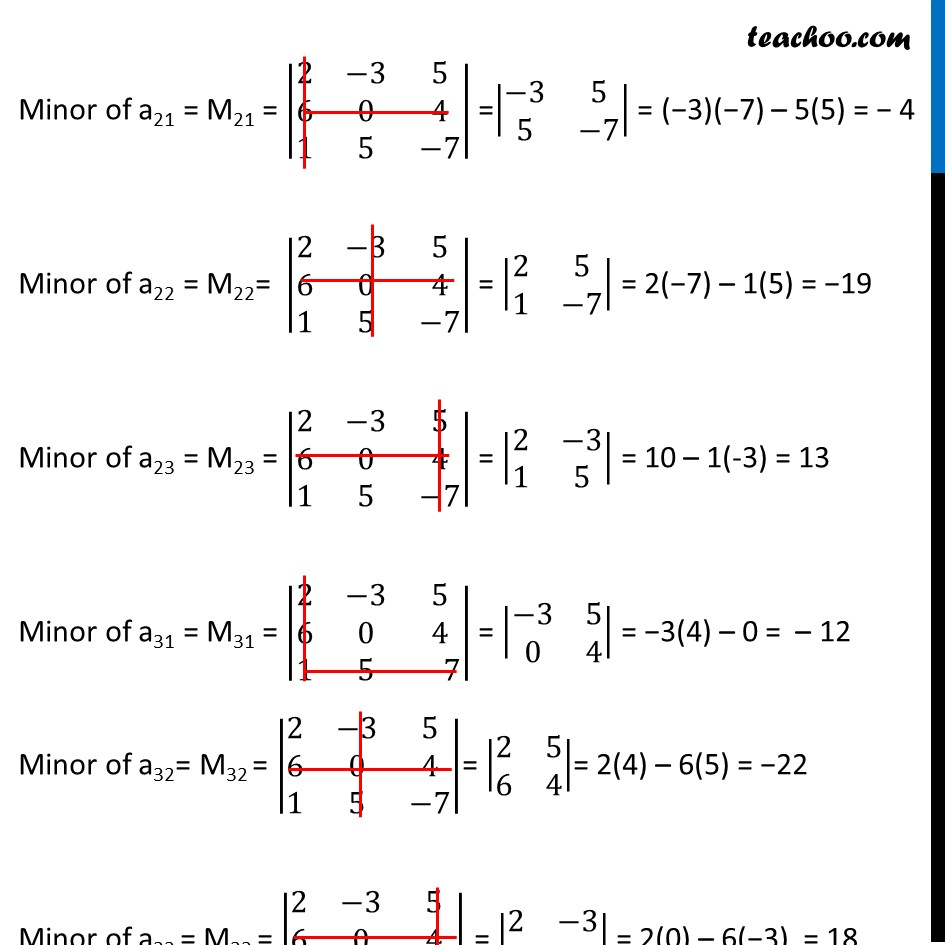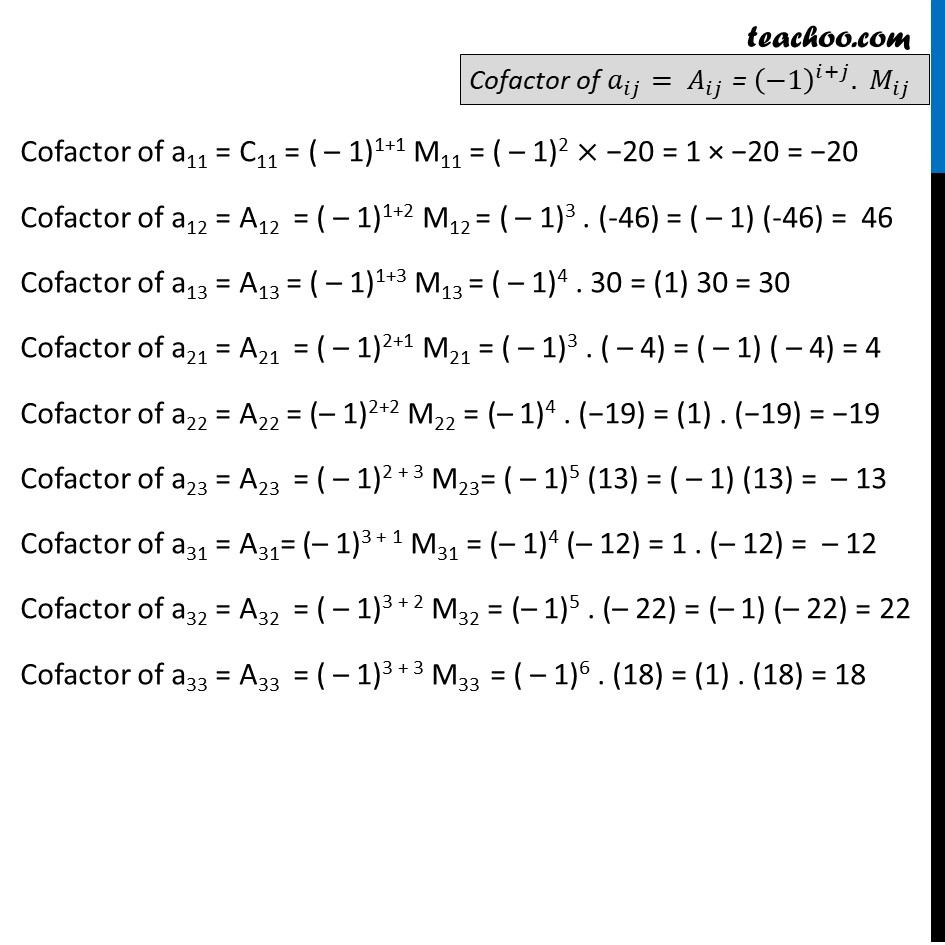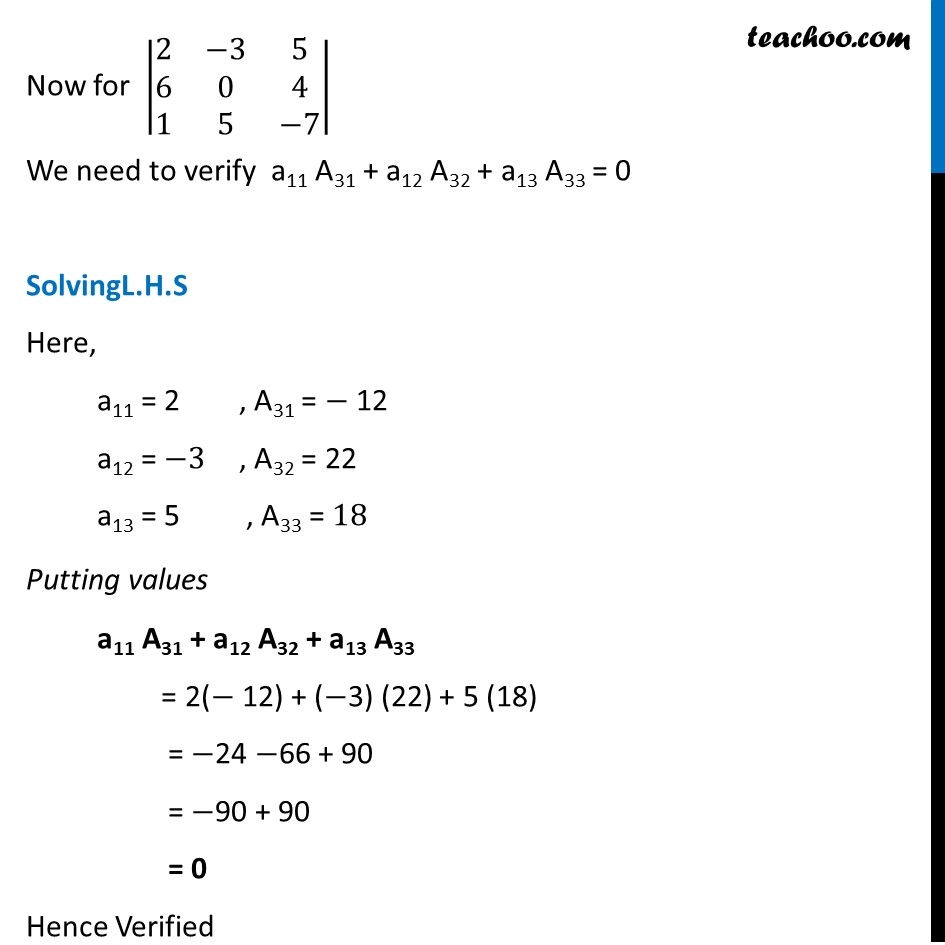Examples

Chapter 4 Class 12 Determinants
Serial order wiseLearn in your speed, with individual attention - Teachoo Maths 1-on-1 Class

### Transcript

Example 11 Find minors and cofactors of the elements of the determinant |■8(2&−3&5@6&0&4@1&5&−7)| and verify that a11 A31 + a12 A32 + a13 A33 = 0 First, finding minors and cofactors Minor of a11 = M11 = |■8(2&−3&5@6&0&4@1&5&−7)| = |■8(0&4@5&−7)| = 0 – 5(4) = −20 Minor of a12 = M12 =|■8(2&−3&5@6&0&4@1&5&−7)| = |■8(6&4@1&−7)| = 6(-7) – 1(4) = − 46 Minor of a13 = M13 = |■8(2&−3&5@6&0&4@1&5&−7)| = |■8(6&0@1&5)| = 6(5) – 0 = 30 Minor of a21 = M21 = |■8(2&−3&5@6&0&4@1&5&−7)| =|■8(−3&5@5&−7)| = (−3)(−7) – 5(5) = − 4 Minor of a22 = M22= |■8(2&−3&5@6&0&4@1&5&−7)| = |■8(2&5@1&−7)| = 2(−7) – 1(5) = −19 Minor of a23 = M23 = |■8(2&−3&5@6&0&4@1&5&−7)| = |■8(2&−3@1&5)| = 10 – 1(-3) = 13 Minor of a31 = M31 = |■8(2&−3&5@6&0&4@1&5&−7)| = |■8(−3&5@0&4)| = −3(4) – 0 = – 12 Minor of a32= M32 = |■8(2&−3&5@6&0&4@1&5&−7)|= |■8(2&5@6&4)|= 2(4) – 6(5) = −22 Minor of a33 = M33 = |■8(2&−3&5@6&0&4@1&5&−7)| = |■8(2&−3@6&0)| = 2(0) – 6(−3) = 18 Cofactor of a11 = C11 = ( – 1)1+1 M11 = ( – 1)2 × −20 = 1 × −20 = −20 Cofactor of a12 = A12 = ( – 1)1+2 M12 = ( – 1)3 . (-46) = ( – 1) (-46) = 46 Cofactor of a13 = A13 = ( – 1)1+3 M13 = ( – 1)4 . 30 = (1) 30 = 30 Cofactor of a21 = A21 = ( – 1)2+1 M21 = ( – 1)3 . ( – 4) = ( – 1) ( – 4) = 4 Cofactor of a22 = A22 = (– 1)2+2 M22 = (– 1)4 . (−19) = (1) . (−19) = −19 Cofactor of a23 = A23 = ( – 1)2 + 3 M23= ( – 1)5 (13) = ( – 1) (13) = – 13 Cofactor of a31 = A31= (– 1)3 + 1 M31 = (– 1)4 (– 12) = 1 . (– 12) = – 12 Cofactor of a32 = A32 = ( – 1)3 + 2 M32 = (– 1)5 . (– 22) = (– 1) (– 22) = 22 Cofactor of a33 = A33 = ( – 1)3 + 3 M33 = ( – 1)6 . (18) = (1) . (18) = 18 Now for |■8(2&−3&5@6&0&4@1&5&−7)| We need to verify a11 A31 + a12 A32 + a13 A33 = 0 SolvingL.H.S Here, a11 = 2 , A31 = − 12 a12 = −3 , A32 = 22 a13 = 5 , A33 = 18 Putting values a11 A31 + a12 A32 + a13 A33 = 2(− 12) + (−3) (22) + 5 (18) = −24 −66 + 90 = −90 + 90 = 0 Hence Verified